# Abacus

Abacus

Did you know that the Cranmer Abacus is specially designed for blind people to operate?

An abacus helps us in understanding mathematical concepts by developing number sense, something that cannot always be achieved while using a calculator.

##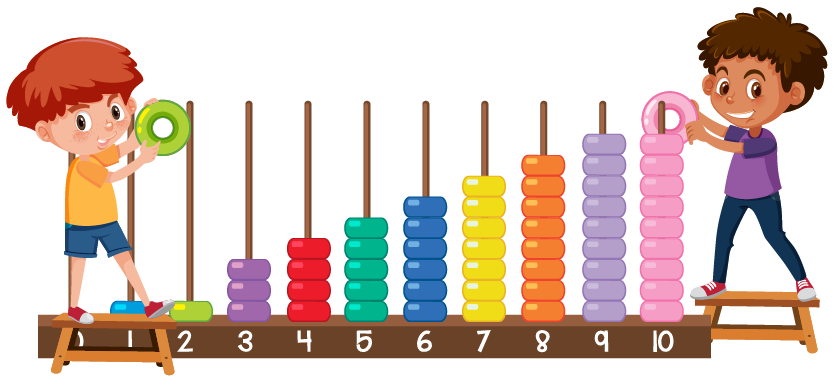In this mini-lesson, we will learn about the abacus - how to use, abacus calculator, and learning abacus as we learn the concept of the abacus.

## Lesson Plan

 1 What Is Abacus? 2 Important Notes on Abacus 3 Solved Examples on Abacus 4 Challenging Questions on Abacus 5 Interactive Questions on Abacus

## What Is Abacus?

An abacus is a simple calculating device that consists of beads arranged in rods of wires embedded in a wooden frame.

Calculations can be performed by sliding beads along the rods.

### Parts of Abacus

The different parts of an abacus are:

• The outer boundary of the abacus is called the frame.
• The abacus we use currently has 17 rods and each rod has 5 beads.
• There are two divisions in an abacus. The horizontal bar that divides the upper and lower part is called the beam.
• The beads that are in the lower part are called the lower beads or the earth beads.
• The beads that are in the upper part are called the upper beads or the heaven beads.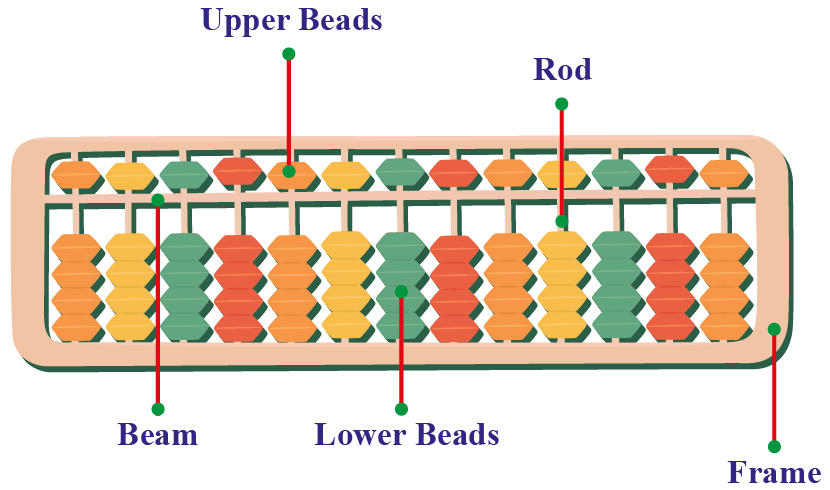## How to Count Numbers on Abacus?

An abacus is a device that can be used to count, add, subtract, multiply, and more.

• The column that is on the extreme right is for the one's place.
• The next column is used for the ten's place.
• The third column from the right end is for the hundred's place and so on.

### Abacus - How to Use

Counting on an abacus is done following the decimal system.

Abacus is generally split into two rows. The top row is 5 times the bottom row. After 5 lower beads are counted, the result is "carried" to the upper row.

Examples

1. Counting 532 using abacus:

• Slide 2 beads up on the right-most column.
• 3 beads up on the $$2^{ \text {nd}}$$ column from the right.
• 5 beads up on the $$3^{ \text {rd}}$$ column from the bottom right corner.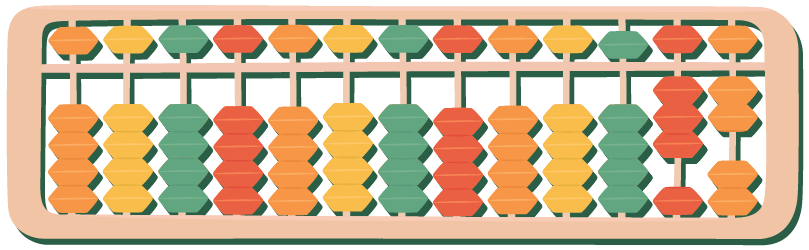2. Counting 8 using the top and bottom row:

• Use 1 bead from the top row which is equivalent to 5 and 3 beads from the bottom row.
• The calculation would thus give 5 + 3 = 8 as the answer.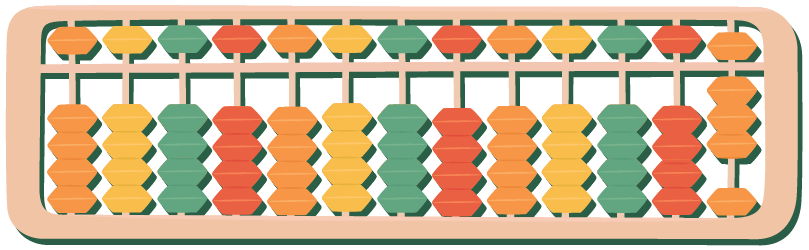The following table shows different abacus like calculating devices used in different countries:

Country Device
China Suanpan
Japan Soroban
Russia Tchoty

## How to Add and Subtract Using Abacus?

Once you know how to use an abacus for counting, you can use it for multiple other operations.

#### The 10 Strategy

If we have to add 8 + 6, we will enter 6 and 8 in the first two columns.

Then move from 6 to 8 so that 8 becomes 10 and 6 becomes 4.

So now we can easily operate 10 + 4 = 14.

Once you master this strategy on an abacus, you can try doing this mentally.

The Two 5s Strategy

If we have to add 6 + 8, we will enter 6 and 8 in the first two wires. The two 5s will make it 10, and we will remain with 4 beads. Now performing 10 + 4 = 14 is easy.

This strategy works in problems where the two numbers being added are more than five.

Suppose we have to add 57 + 86. We will have to represent 86 on the abacus.

The first wire from the right will have 6 and the second wire will have 8. Start with the first wire and add 7 to 6. This will result in 13.

Keep the digit 3 and pass on 1 to 8, thereby making it 9.

Now perform 9 + 5 which will result in 14.

So, the actual result will be 143.

### Subtraction

The process of subtraction is the opposite of addition.

We simply need to borrow the digits from the previous column instead of carrying them over.

Example

If we want to subtract 672 from 945,

• Enter 945 in the abacus and start subtracting column by column from the left.
• If we subtract 2 from 5, we will get 3 in the one's place.
• Follow a similar method for ten's places. Since we cannot subtract 7 from 4, we will have to borrow 1 from the hundred's place, leaving 8 in the hundred's place. Now we will get 14 in the ten's place. Subtracting 7 from 14 we get 7 in the ten's place.
• Finally, in the hundred's place, we will subtract 6 from 9 and thus will be left with 3 beads.

So our final answer will be 945 - 672 = 273.

### Abacus Calculator

Let us try to solve mathematical operations using the following abacus calculator:

## What Are the Advantages of Using Abacus?

Using an abacus:

• Boosts mental arithmetic calculations
• Increases concentration
• Sharpens memory
• Improves imagination
• Boosts confidence
• Increases problem-solving skills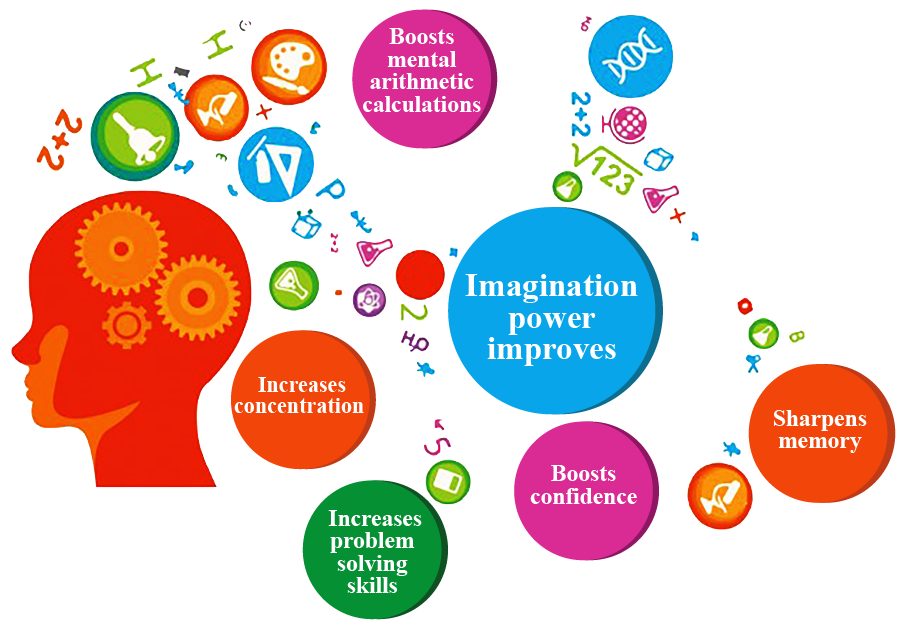## Solved Examples

 Example 1

Can you help Martha represent the number 3687 in an abacus?

Solution

Number 3687 can be represented in an abacus like this: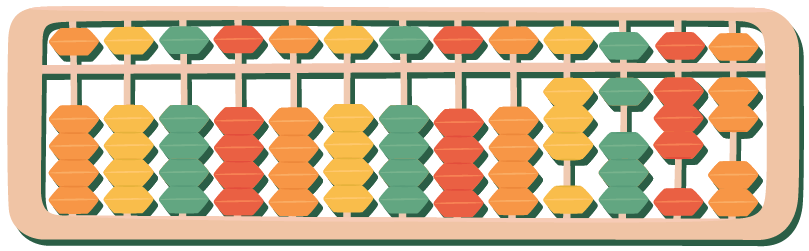• Slide 2 beads up on the lower row and 1 bead down on the upper row in the right-most column.
• 3 beads up on the lower row and 1 bead down on the upper row in the $$2^{ \text {nd}}$$ column from the right.
• 1 bead up on the lower row and 1 bead down on the upper row in the $$3^{ \text {rd}}$$ column from the right.
• Slide 3 beads up in the $$4^{ \text {th}}$$ column from the right.
 Example 2

Lizzy is stuck in a problem while adding two numbers. Can you help her by writing down the steps to add 456 and 796 using an abacus?

Solution

The steps to calculate the sum of  456 and 796:

• We will have to represent 796 on the abacus.
• The first wire from the right will have 6, the second wire 9, and the third wire 7.
• Start with the first wire and add 6 to 6. This will result in 12. Keep the digit 2 and pass on 1 to 9, thereby making it 10.
• Now perform 10 + 5 which will result in 15. Keep 5 and carry 1 forward to the next wire.
• Now, add 8 (7 + 1) and 4, which will give 12 as the result.

So, the result will be 1252.

 $$\therefore$$ Sum = 1252
 Example 3

Mike is learning to represent numbers on an abacus. Can you tell him which number is represented in the following abacus: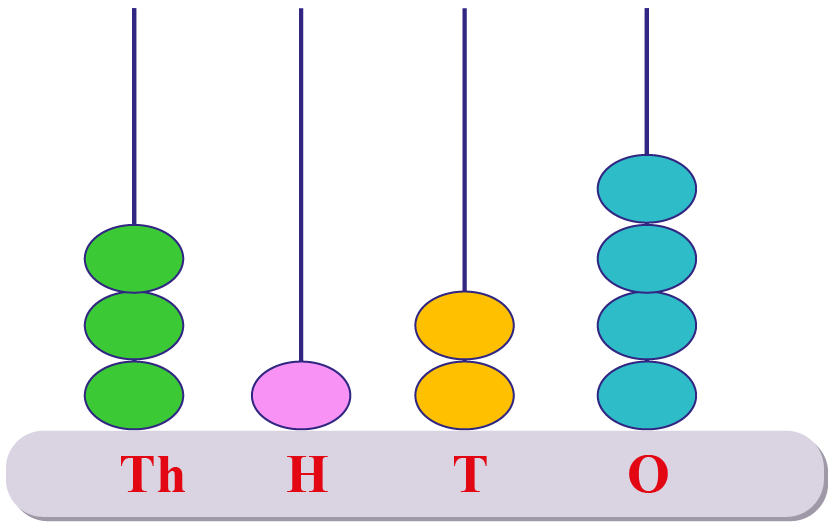Solution

The beads represented on the abacus are in the following fashion:

• 4 in one's place
• 2 in ten's place
• 1 in hundred's place
• 3 in hundred's place

So the number represented = 3124.

 $$\therefore$$ Number represented = 3124
 Example 4

What are the operations that can be done on an abacus?

Solution

The various operations that can be performed using an abacus are:

• Subtraction
• Division
• MultiplicationChallenging Questions
• How would you use an abacus to perform multiplication and division?

## Interactive Questions

Here are a few activities for you to practice. Select/Type your answer and click the "Check Answer" button to see the result.

## Let's Summarize

The mini-lesson targeted the fascinating concept of the abacus. The math journey around abacus starts with what a student already knows, and goes on to creatively crafting a fresh concept in the young minds. Done in a way that is not only relatable and easy to grasp, but will also stay with them forever. Here lies the magic with Cuemath.

At Cuemath, our team of math experts is dedicated to making learning fun for our favorite readers, the students!

Through an interactive and engaging learning-teaching-learning approach, the teachers explore all angles of a topic.

Be it worksheets, online classes, doubt sessions, or any other form of relation, it’s the logical thinking and smart learning approach that we, at Cuemath, believe in.

## 1. What is the full form of abacus?

The full form of the abacus is the Abundant Beads, Addition, and Calculation Utility System.

It is a simple calculating device that consists of beads arranged in rods of wires embedded in a wooden frame.

## 2. How is abacus useful in mathematics?

Abacus is a device that can be used to perform mathematical operations like addition, subtraction, multiplication, and division.

Thus it helps reducing time while doing calculations in mathematics with greater efficiency.

## 3. How do you represent numbers in an abacus?

An abacus is a device that can be used to count, add, subtract, multiply, and more.

The numbers can be represented as:

• The column that is on the extreme right is for the one's place.
• The next column is used for the ten's place.
• The third column from the right end is for hundred's place and so on.
More Important Topics
Numbers
Algebra
Geometry
Measurement
Money
Data
Trigonometry
Calculus
More Important Topics
Numbers
Algebra
Geometry
Measurement
Money
Data
Trigonometry
Calculus
Learn from the best math teachers and top your exams

• Live one on one classroom and doubt clearing
• Practice worksheets in and after class for conceptual clarity
• Personalized curriculum to keep up with school